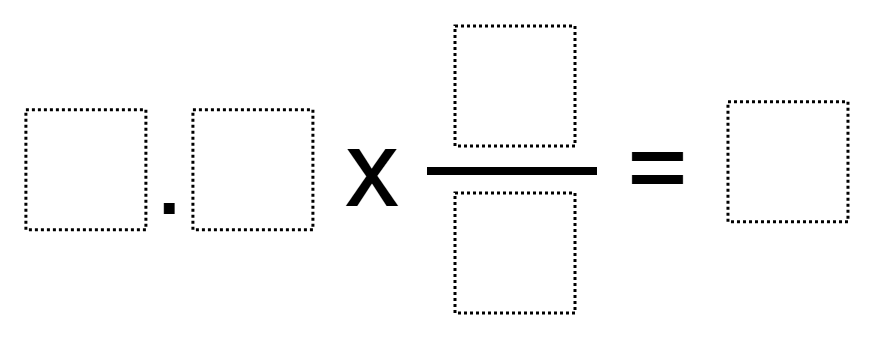# Multiplying A Decimal By A Fraction to Get a Whole Number

Directions: Using the digits 1 to 9, at most one time each, fill in the boxes to make a true statement.### Hint

What digits would be a bad choice for the denominator?
What digits would be a good choice for the denominator?

There are multiple answers, but here are 2:

1.5 * 4/2 = 3
And 3.5 * 2/1 = 7

Source: Owen Kaplinsky

## Equivalent Expressions with Fractions

Directions: Using the digits 0 to 9 at most one time each and choosing either …

1.2.3.